## Example Questions

### Example Question #1 : Arithmetic Sequences

Which of the following completes the number sequence 4, 7, 11, 16, __________ ?Explanation:

Sequencing problems require us to look at the numbers given to us ad decipher a pattern.

7  4 = 3 so 3 was added to the first number (4)

11 – 7 = 4 so 4 was added to the second number (7)

16  11 = 5 so 5 was added to the third number (11)

If it is to continue in this pattern, then 6 should be added to 16, yielding 22 as the correct answer

### Example Question #2 : Arithmetic Sequences

Find the 50th term in the following sequence.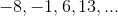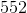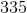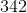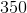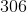Explanation:

A sequence is simply a list of numbers that follow some sort of consistent rule in getting from one number in the list to the next one.  Sequences generally fall into three categories:  arithmetic, geometric, or neither.

In arithmetic sequences, I add the same number each time to get from one number to the next.  In other words, the difference between any two consecutive numbers in my list is the same.

In geometric sequences, I multiply by the same number each time to get from one number to the next.  In other words, the ratio between any two consecutive numbers in my list is the same.

Finally, sequences that are neither, still follow some rule, but it just happens not to be one of these two.

Looking at our sequence, we might quickly notice that each number is simply 7 more than the number before.  In other words, I can find the next number by adding 7 each time.  Hence, our sequence is arithmetic.

Unfortunately, we need to find the 50th term in this sequence, and the problem only got us through the first four.  A simple (yet way too time-consuming approach) would be to keep adding 7 until we get to term number 50.  Not only is that the long way, we also risk losing count and ending up on the wrong term.  So what's the easier way?

The easier way hinges on the fact that I am simply adding 7 over and over again.  If I want to find the 2nd term, I start with the 1st term and add 7 once.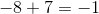To find the 3rd term, I add 7 twice.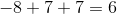You might already see the pattern.  For the 4th term I would add 7 three times, for the 5th four times, 6th five times, etc.

Notice that to find any term, I simply add 7 one less time than the number of the term.  Therefore, to find the 50th term, I would add 7 forty-nine times.

But adding 7 forty-nine times is the same as adding forty-nine 7s.  But forty-nine 7s are the same as 49 times 7.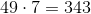Therefore, to find the 50th term, I simply need to add 343 to our starting value.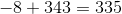### Example Question #3 : Arithmetic Sequences

What is the next term in the sequence: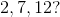Explanation:

Because it is an arithmetic sequence, the difference between each term is the same. Therefore find out the difference between any two consecutive terms.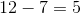and so the sequence increases by 5 each term. Thus the answer is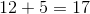.

### Example Question #4 : Arithmetic Sequences

The sum of four consecutive integers is 42. What is the value of the greatest number?

12

14

15

11

13

12

Explanation:

We can represent four consecutive integers using the following expressions:Create an equation using the information in the problem.Subtract 6 from both sides of the equation.Divide both sides of the equation by 4.The highest number in the series is the x-variable plus 3. We can write the following: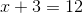.

### Example Question #5 : Arithmetic Sequences

Which of the following numbers completes the sequence 40, 33, 27, 22, 18, 15, ...?

14

13

16

12

7.5